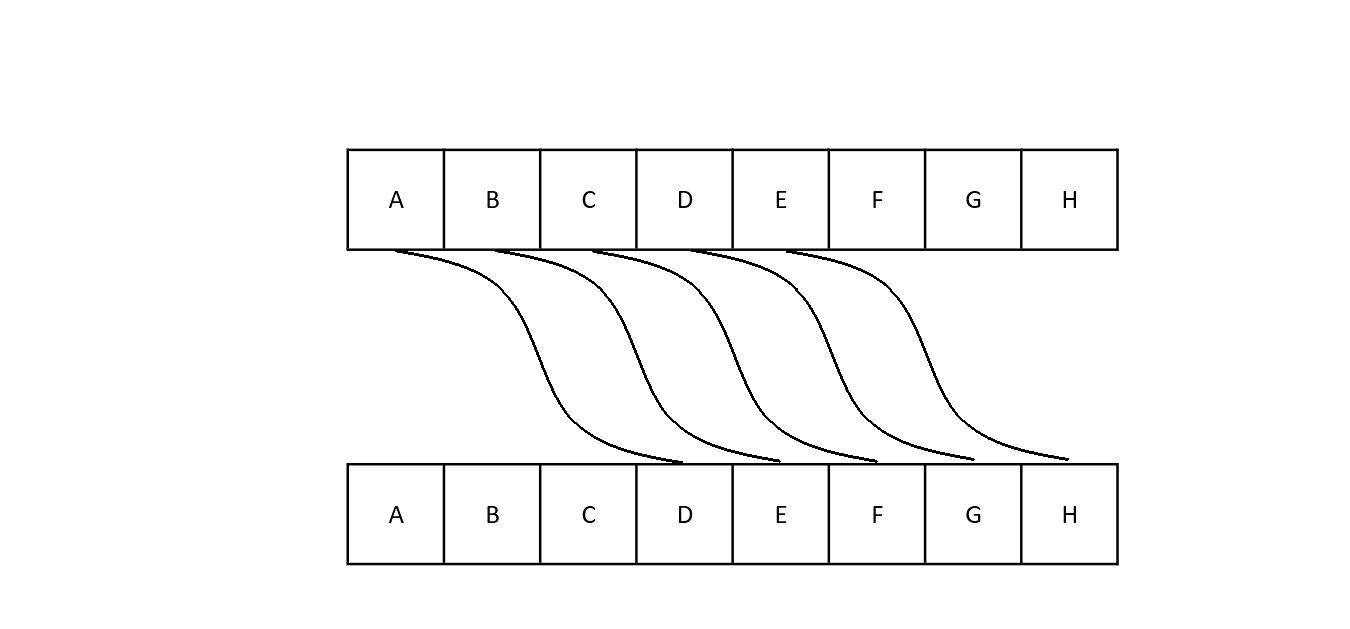# Substitution Cipher

## Introduction¶

Substitution cipher is an encryption scheme, in which position of plaintext units are altered, keeping the sequence same. Basically it means that each character of the message is substituted with a different character to make the ciphertext It is the oldest and simplest way of encrypting data.

Some popular examples of substitution ciphers are: Caesar cipher, ROT13 etc

### Caesar cipher¶

Caesar cipher is one of the oldest and simplest method used for secret communication. The cipher is named after Julius Caesar who used it to send secret messages to his generals. It is a monoalphabetic cipher which means a single character is encrypted at a time. It is also a shift cipher which means that each letter of the plaintext is shifted by a fixed number down the alphabet to get the corresponding ciphertext. So if alphabet ‘a’ is to be encrypted using key 3 then it will be encrypted as, ’a’ + 3 = ‘d’.

Here's a diagrammatic representation of the same:Let us understand the concept better using this example ,

Suppose Alice and Bob want to send messages to each other through an insecure channel. They wanted to use the “simplest” way of encrypting the messages so they agreed upon using Caesar cipher. Hence Alice sets a key of 3. She wants to send “HELLO”, so she replaces each alphabet with the corresponding 4th alphabet that is, ‘H’ -> ‘K’ , ‘E’ -> ‘H’ , ‘L’ -> ‘O’ , ‘L’ -> ‘O’ , ‘O’ -> ‘R’

Finally, plaintext: “HELLO” → ciphertext: “KHOOR”

To decrypt the message Bob is going to backshift the ciphertext with the given key. As no one else has the key, no one can know the message and hence security is ensured. But this is not true in the present world. As the key length is limited to maximum of 26. Thus, breaking the caesar cipher becomes easier. It can be brute forced easily to find the actual message. Also the repetition of same alphabets makes patterns and gives the attacker a clue to break it.

ROT13

ROT13 is a special case in Caesar cipher. ROT13 stands for “rotate by 13” i.e it always replaces each plaintext character with the corresponding 13th alphabet. To put it simply it is a case of caesar cipher where the key is taken as 13. You can see that as 13 is the half of 26, it made to sense to some to take the key as 13, which is most distant from 0 or 26. One can get the plaintext by re-doing the same operation as explained above. Even ROT13 isn’t secure like Caesar cipher. It can be broken easily. ROT13 was earlier used in net.jokes newsgroup in the early 1980s.

ROT13(ROT13(X)) == X


For example, ‘M’ -> ‘Z’ , ‘I’ -> ‘V’ , ‘D’ -> ‘Q’ , ‘D’ -> ‘Q’ , ‘L’ -> ‘Y’ , ’E’->’R’

Finally, plaintext: ”MIDDLE” → ciphertext: “ZVQQYR”